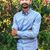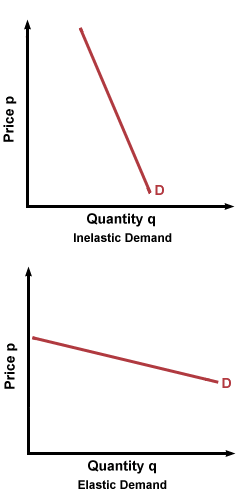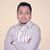# Product price increase to increase the revenue of firm, what do you think?

A manager wants to increase revenue of his firm. Should he raise the price of his product? (discuss)

This post was part of TyroCity discussion forumRevenue of a firm refers to the income generated by it through the sale of goods and services at different prices. In other words, revenue is a money that a firm earns from selling its product. The revenue concepts are concerned with Total Revenue (TR), Average Revenue (AR) and Marginal Revenue (MR). Revenue can be calculated (Guell, 2012). Revenues equal the number of units that a firm sells times the price at which it sells each unit:

Revenues = price × quantity.If a firm increases the price of his product then it will not definitely increase the revenue of a firm because the increase in total revenue of a firm always depends upon the nature of the elasticity of demand.

A manager of a firm should always has to understand the elasticity of demand for increasing the revenue of his firm because demand elasticity is the crucial factor that determines how a price increase will affect the firm’s revenue A manager should always consider two things i.e. the elastic demand and inelastic demand to increase the revenue of a firm.

Elastic Demand

If a consumer is price sensitive then the demand is elastic. If a firm increase the price of a product it will lead to a large reduction in volume. In other words, the increase in price leads to a decrease in the quantity demanded. Thus, in this case, a firm has to keep its price low.

Inelastic Demand

If consumers do not price sensitive then the demand is regarded as inelastic where raising the product’s price will lead to a small reduction in quantity. In other words, the increase in the price leads to an increase in quantity demanded. Thus, in this case, a firm can increase its product price to increase revenue.

In conclusion, the revenue of a firm depends upon the elasticity of demand and a manager should always consider the nature of demand elasticity before setting up the price to increase revenue.

References
Guell, R. C. (2012). Issues in Economics Today (Six ed.). New York: McGraw-Hill.Guell (2015), in their book Issues in Economics Today, elasticity is a measure of how much buyers and sellers respond to changes in market conditions. It allows us to analyze supply and demand with greater accuracy. Here, a manager wants to increase revenue of his firm. The reason how the he would increase revenue is explained below:

Reason I - elastic demand curve

With an elastic demand curve, an increase in the price leads to a decrease in quantity demanded that is proportionately larger. Thus, total revenue decreases.

Figure 1: When demand is elastic

Illustration:

Ep = dQ/dP * P/Q
= (12-8)/(200-250) * (250+200)/(8+12)
= - (180/100)
= - 1.8
= 1.8 > 1, elastic

At, combination A, Total Revenue = 200 * 12 = Rs. 2400

At, combination B, Total Revenue = 250 * 8 = Rs. 2000

Hence, if the demand of product is elastic then the manger should not raise the price in order to increase firms’ revenue.

Reason II - inelastic demand curve

With an inelastic demand curve, an increase in the price leads to an increase in quantity demanded that is proportionately larger. Thus, total revenue increases.

Figure 2: When demand is inelastic

Illustration:

Ep = dQ/dP * P/Q
= - 0.81
= 0.81 < 1, inelastic

At, combination A, Total Revenue = 200 * 12 = Rs. 2400

At, combination B, Total Revenue = 250 * 10 = Rs. 2500

Hence, if the demand of product is inelastic then the manger should raise the price in order to increase firms’ revenue.

To state more precisely, the concept of price elasticity of demand is highly applicable for managers of the company at the time of make pricing decision of their firm’s products and services. The core concept of it depicts that if the product of the company is inelastic then the manager can increase the price in order to increase the revenue of the company. By increasing the price of the product the revenue can be raised. Similarly, if the product appears to be elastic then it would be better that manger does not increase the price of the product.

Reference

Guell, Robert C. (2015). Issues in Economics Today , 7th edition- 2015 ISBN: 978-0078021817